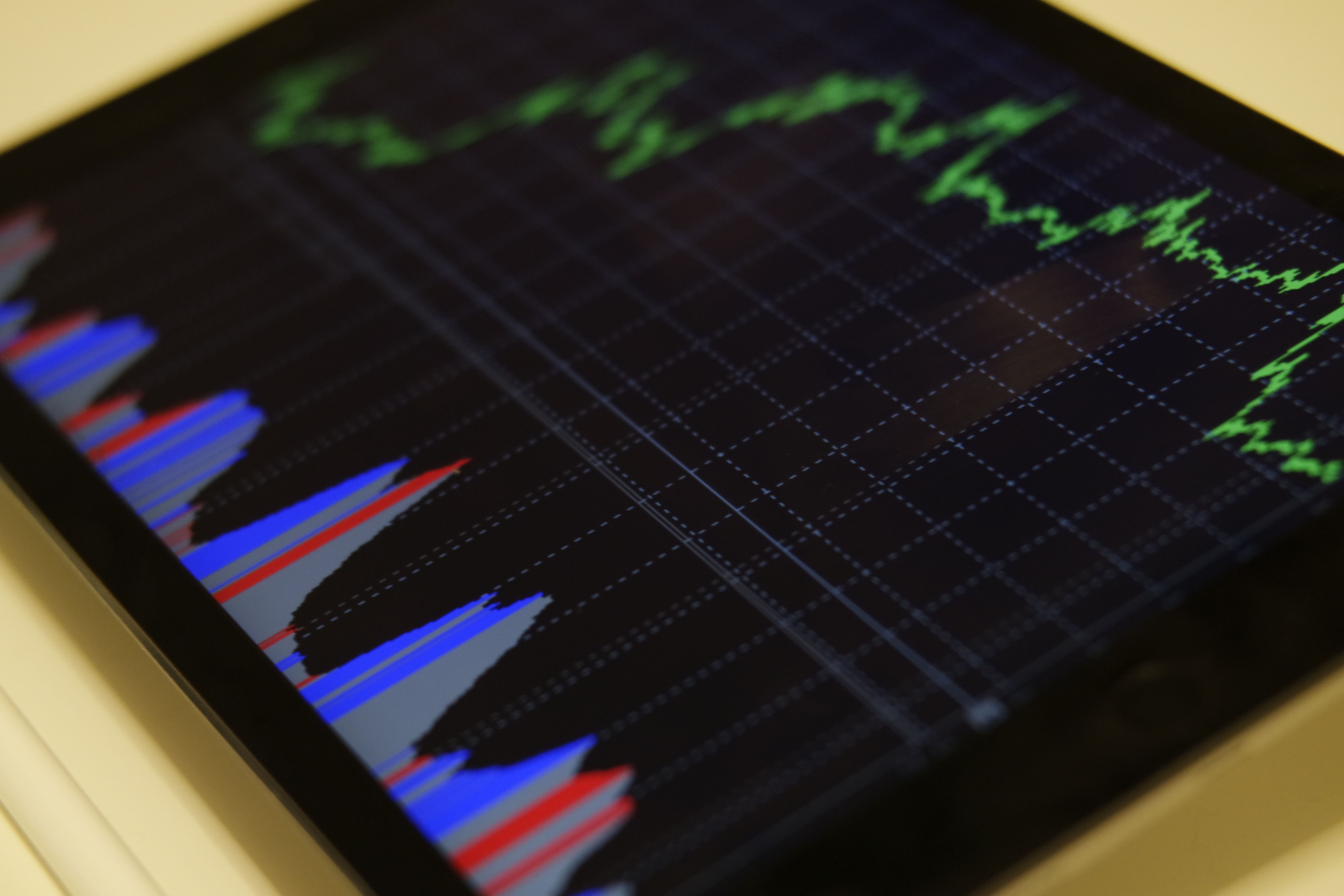# Financial Ratio Analysis Tutorial Part 2 – The Quick Ratio (Acid Test Ratio) – Definition & Formula

the quick ratio also known as the acid test ratio is a measure of liquidity that describes how well a company could pay off its current liabilities with these current assets the quick ratio is a more conservative measure than the current ratio because it excludes Emmet's away from its calculation the quick ratio can be defined as current assets minus inventory divided by current liabilities there are even more refined versions of the quick ratio that exclude all current assets and only consider the most liquid and current assets it is important to make sure we're comparing financial ratios that the results for both ratios or the result of the same moment another formula for the quick ratio is cash and cash equivalents plus short-term investments plus accounts receivables divided by current liabilities generally higher ratios better higher ratios mean that the company is less likely to experience financial distress and all current liabilities were to come due also lenders such as banks look at liquidity ratios to make decisions about loans the higher the quick ratio the more likely a company is to get approved for low-interest loans that it can be used to make profitable investments however just because one company has a higher quick ratio than another does not always mean that it is a better company a company could just keep all of its earnings in cash and Billups liquidity ratios but this would be at the expense of the owners of these earnings were not paid out as dividends or invested in profitable as with anything else there needs to be balance now we'll take a look at the balance sheet for Pier one Imports and Bed Bath and Beyond to calculate their quick ratios and compare them to each other first we'll find the quick ratio from Pier one Imports to do this we need to get their most recent balance sheet which can be found their annual report which can be found on their website under investor relations as you can see here ones total current assets are 650 million three hundred and fourteen thousand dollars I've watched three hundred and twenty-two million four hundred and eighty two thousand dollars his inventories and their total current liabilities are 245 million three hundred eighty eight thousand dollars with this information we can calculate the quick ratio by plugging these numbers into the formula here ones quick ratio is one point three three six now let's find the quick ratio for Bed Bath and Beyond just as we did with Pier one we need to find Bed Bath & Beyond most recent annual report to get the most recent annual balance sheet this can be found on their company website under investor relations as you can see at Beth Leon's total current assets are four billion one hundred and forty two million nine hundred and thirty nine thousand dollars of which two billion 71 million eight hundred ninety thousand dollars of this merchandise inventory and bed bath Allianz total current liabilities are 1 billion three hundred and thirty nine million one hundred and thirty thousand dollars we plug these numbers into the quick ratio formula and we find the quick ratio is one point five four six there is a significant difference if we were to compare both the company's current ratios to their quick ratios this is because inventory makes up about 50 percent of both company's total current assets this shows the significance of how much more conservative the quick ratio is than the current ratio if either Pier one Imports or Bed Bath and Beyond inventory is not highly marketable or not worth the amounts reported on the balance sheet the current ratio would have painted an unrealistic picture since the quick ratio excludes inventory we can also exclude any uncertainties concerning inventory however there is still a flaw the quick ratio sensitive factors and accounts receivables if two companies had identical balance sheets except for one company collected its accounts receivable faster than the other then even though their ratios would be the same the company that collects us perceivable is faster would actually be the most liquid while the current ratio and quick ratio theoretically makes sense investors need to be aware of the flaws and understand that the true measure of liquidity takes into account the true amount of time it takes for a company to convert its working capital assets into cash